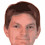## Friday, 10 December 2010

### Computus 1

Computus is the calculation of Easter. Calendrical calculations being a bit of an obsession of mine, I've been looking at this with a view to an implementation using limited-range integer arithmetic. There's a lot of interesting stuff still being researched on this, but I haven't seen a good implementation for 16-bit unsigned arithmetic. So here's my attempt:

void easter_u16(u16 year, u16* month, u16* day)
{
u16 a = year % 19;
u16 b = year >> 2;
u16 c = (b / 25) + 1;
u16 d = (c * 3) >> 2;
u16 e = ((a * 19) - ((c * 8 + 5) / 25) + d + 15) % 30;
e += (29578 - a - e * 32) >> 10;
e -= ((year % 7) + b - d + e + 2) % 7;
d = e >> 5;
*day = e - d * 31;
*month = d + 3;
}

This is valid for all Gregorian years 0 to 65535. In fact, the core algorithm is valid for all non-negative years: just change the integer type to a wider, signed one.

If you want to test it using Dr J R Stockton's wonderful script tester, use the following Javascript snippet:

var a = YR % 19;
var b = YR >>> 2;
var c = ((b / 25)|0) + 1;
var d = (c * 3) >>> 2;
var e = ((a * 19) - (((c * 8 + 5) / 25)|0) + d + 15) % 30;
e += (29578 - a - (e << 5)) >>> 10;
e -= ((YR % 7) + b - d + e + 2) % 7;
return e;

1.It would, I think, be well to state what authority you are using to define Easter Sunday - directly from the Calendar Act or Prayer Book,
directly from Gregory XIII and Clavius, or by way of an intermediate. JRS.

2.Indeed! It's Gregorian Easter according to the Book of Common Prayer. The starting point for my conversion to 16-bit arithmetic was the 'EasterJRS()' JavaScript function from 'http://www.merlyn.demon.co.uk/estr-inc.js'

3.Hi Ian,

May I port this implementation to an open-source Java project and release it for other developers to use?

4.5.